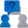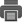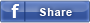• Enquiry

# ELECTROMAGNETIC BALANCE

CAT NO: DIDACTICELECTROMETER0133

### Description & Specification:

ELECTROMAGNETIC BALANCE.
ELECTROMAGNETIC BALANCE The end of one of the two arms of this balance is equipped with a rectangular turn of aluminium with base of 4 cm, immersed in the field of a powerful permanent magnet. The other arm is provided with two sliding masses that enable to reach the equilibrium in rest conditions. When some current crosses the circuit, a force F is generated between magnetic field B and electric current I : its value is determined by Ampère’s law: F = B • l • I • sine α where l is the length of the conductor and α is the angle existing between the conductor and the magnetic fi eld. Thus it is possible to verify that the intensity of this force is maximum when α = 90°, and null when α = 0°. Value I of electric current can be read on the ammeter included in the equipment ; then induction B of the permanent magnet can be determined. Repeat this test by replacing the permanent magnet with a solenoid. Thus the relation that gives the value of the magnetic field inside a solenoid can be verified. Balance sensitivity: 10 mg Dimensions : 45 x17 x 22 cm. COMPONENTS: • 1 Balance elettromagnetica • 1 permanent magnet • 1 Solenoid • 1 Set of weights of 200 g with fractions of gram • 1 Heat sink resistorAdd to Wish List